VHDL coding tips and tricks: Non-synthesisable VHDL code for 8 point FFT algorithm

## Monday, June 27, 2011

### Non-synthesisable VHDL code for 8 point FFT algorithm

A Fast Fourier Transform(FFT) is an efficient algorithm for calculating the discrete Fourier transform of a set of data. A DFT basically decomposes a set of data in time domain into different frequency components. DFT is defined by the following equation:A FFT algorithm uses some interesting properties of the above formula to simply the calculations. You can read more about these FFT algorithms here.
Many students have been asking doubts regarding vhdl implementation of FFT, so I decided to write a sample code. I have selected 8 point decimation in time(DIT) FFT algorithm for this purpose. In short I have wrote the code for this flow diagram of FFT.
This is just  a sample code, which means it is not synthesisable. I have used real data type for the inputs and outputs and all calculations are done using the math_real library. The inputs can be complex numbers too.
To define the basic arithmetic operations between two complex numbers I have defined some new functions which are available in the package named fft_pkg. The component named, butterfly , contains the basic butterfly calculations for FFT as shown in this flow diagram.
I wont be going any deep into the theory behind FFT here. Please visit the link given above or Google  for in depth theory. There are 4 vhdl codes in the design,including the testbench code, and are given below.

The package file - fft_pkg.vhd:

library IEEE;
use IEEE.std_logic_1164.all;
use IEEE.MATH_REAL.ALL;

package fft_pkg is

type complex is
record
r : real;
i : real;
end record;

type comp_array is array (0 to 7) of complex;
type comp_array2 is array (0 to 3) of complex;

function add (n1,n2 : complex) return complex;
function sub (n1,n2 : complex) return complex;
function mult (n1,n2 : complex) return complex;

end fft_pkg;

package body fft_pkg is

--addition of complex numbers
function add (n1,n2 : complex) return complex is

variable sum : complex;

begin
sum.r:=n1.r + n2.r;
sum.i:=n1.i + n2.i;
return sum;
end add;

--subtraction of complex numbers.
function sub (n1,n2 : complex) return complex is

variable diff : complex;

begin
diff.r:=n1.r - n2.r;
diff.i:=n1.i - n2.i;
return diff;
end sub;

--multiplication of complex numbers.
function mult (n1,n2 : complex) return complex is

variable prod : complex;

begin
prod.r:=(n1.r * n2.r) - (n1.i * n2.i);
prod.i:=(n1.r * n2.i) + (n1.i * n2.r);
return prod;
end mult;

end fft_pkg;

The top level entity - fft8.vhd:

library IEEE;
use IEEE.STD_LOGIC_1164.ALL;
use IEEE.MATH_REAL.ALL;
library work;
use work.fft_pkg.ALL;

entity fft8 is
port(   s : in comp_array; --input signals in time domain
y : out comp_array  --output signals in frequency domain
);
end fft8;

architecture Behavioral of fft8 is

component butterfly is
port(
s1,s2 : in complex;      --inputs
w :in complex;      -- phase factor
g1,g2 :out complex      -- outputs
);
end component;

signal g1,g2 : comp_array := (others => (0.0,0.0));
--phase factor, W_N = e^(-j*2*pi/N)  and N=8 here.
--W_N^i = cos(2*pi*i/N) - j*sin(2*pi*i/N);  and i has range from 0 to 7.
constant w : comp_array2 := ( (1.0,0.0), (0.7071,-0.7071), (0.0,-1.0), (-0.7071,-0.7071) );

begin

--first stage of butterfly's.
bf11 : butterfly port map(s(0),s(4),w(0),g1(0),g1(1));
bf12 : butterfly port map(s(2),s(6),w(0),g1(2),g1(3));
bf13 : butterfly port map(s(1),s(5),w(0),g1(4),g1(5));
bf14 : butterfly port map(s(3),s(7),w(0),g1(6),g1(7));

--second stage of butterfly's.
bf21 : butterfly port map(g1(0),g1(2),w(0),g2(0),g2(2));
bf22 : butterfly port map(g1(1),g1(3),w(2),g2(1),g2(3));
bf23 : butterfly port map(g1(4),g1(6),w(0),g2(4),g2(6));
bf24 : butterfly port map(g1(5),g1(7),w(2),g2(5),g2(7));

--third stage of butterfly's.
bf31 : butterfly port map(g2(0),g2(4),w(0),y(0),y(4));
bf32 : butterfly port map(g2(1),g2(5),w(1),y(1),y(5));
bf33 : butterfly port map(g2(2),g2(6),w(2),y(2),y(6));
bf34 : butterfly port map(g2(3),g2(7),w(3),y(3),y(7));

end Behavioral;

Butterfly component - butterfly.vhd:

library IEEE;
use IEEE.STD_LOGIC_1164.ALL;
library work;
use work.fft_pkg.ALL;

entity butterfly is
port(
s1,s2 : in complex;      --inputs
w :in complex;      -- phase factor
g1,g2 :out complex      -- outputs
);
end butterfly;

architecture Behavioral of butterfly is

begin

--butterfly equations.
g1 <= add(s1,mult(s2,w));
g2 <= sub(s1,mult(s2,w));

end Behavioral;

Testbench code - tb_fft8.vhd:

LIBRARY ieee;
USE ieee.std_logic_1164.ALL;
library work;
use work.fft_pkg.all;

ENTITY tb IS
END tb;

ARCHITECTURE behavior OF tb IS
signal s,y : comp_array;

BEGIN

-- Instantiate the Unit Under Test (UUT)
uut: entity work.fft8 PORT MAP (
s => s,
y => y
);

-- Stimulus process
stim_proc: process
begin
--sample inputs in time domain.
s(0) <= (-2.0,1.2);
s(1) <= (-2.2,1.7);
s(2) <= (1.0,-2.0);
s(3) <= (-3.0,-3.2);
s(4) <= (4.5,-2.5);
s(5) <= (-1.6,0.2);
s(6) <= (0.5,1.5);
s(7) <= (-2.8,-4.2);
wait;
end process;

END;

Copy and paste the above codes into their respective files and simulate. You will get the output in the output signal 'y'. Once again, the code is not synthesisable.

Hope the codes are helpful for you. In case you want a synthesisable version of the codes or want a customized FFT vhdl code then contact me.

#### 41 comments:

1.Hi,
I'm new working with VHDL. I would like to know why this code is not synthesisable???

2.@Miguel : I have used real data types in this code. 'real' type is not synthesisable. You cannot convert the real type into a hardware equivalent circuit. so if we have to synthesis the code we have to use fixed point arithmetic for the data types.

1.how can i synthesize ?

3.Thank you Vipin,

What are other options (in addition to fixed-point) to developing a synthesisable code for 8-point fft???

4.@Maguel : There is no fixed answer to this. When you learn vhdl you will know what kind of constructs are not synthesisable. Just avoid using those.
There are many ways to create a fft code. I suggest you learn vhdl before starting writing a fft code.

5.6.hello vipin can you tell me how to write this program i can not understand exactly you wrote 4 program her so please tell me

7.hello vipin
how does the fixed point arithmetic work in place of real? i tried it n its not workin.Can u please help?

8.when i excuting the fft_pkg.vhd.....compiler shows that fft_pkg is neither an entity nor a configaration....
so what should i do?????????

9.Hello..

The code here is not executing..
Please assist me @ the earliest

10.the input for fft should be of in the form of x={2,3,5,1,1,5,3,2} then how come here the sample inputs are given as s(7) <= (-2.8,-4.2);
pls reply.....

11.Hello Vipin,

Thanks so much for the coding........ they all work fine, i would however ask you if i was to include a ram coding following your code, what would be a suitable model for it?.......

Thanks mate.......

1.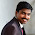hello vipin
please guide me to use package bcuz i have only three options for package..i don't know how to add package..

12.Hey vipin can u suggest me the book or any other resource for learning VHDL language? Please give an early reply.............

13.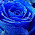hi sir im doing my project related to cordic operator based fft.how can i implement in vhdl.could u pls suggest me as soon as possible.

14.@mayuresh: The Designer’s Guide to VHDL, Peter J. Ashenden is the best book for starters

15.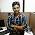hello vipin, the code is giving fatal error at prod.i and prod.r, may be the length mismatch please suggest

1.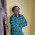i have same dought.. plz help me..

16.i want to implement fft algorithm in vhdl using vedic multiplier as a component. can u help me?

17.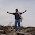Hello...i was trying to implement 32 point FFT but im stucking at declaration of twiddle factors in fixed point please help .....

18.Hello @vipin when i tried this code i get error result in rtl schematics which is shown below , can u sort it out.

WARNING:Xst:1610 - c:/xilinx/bin/web_help_fft_8/dfghj.vhd line 52: Width mismatch. has a width of 1 bits but assigned expression is 2-bit wide.
WARNING:Xst:1610 - c:/xilinx/bin/web_help_fft_8/dfghj.vhd line 53: Width mismatch. has a width of 1 bits but assigned expression is 2-bit wide.
WARNING:Xst:1610 - c:/xilinx/bin/web_help_fft_8/dfghj.vhd line 52: Width mismatch. has a width of 1 bits but assigned expression is 2-bit wide.
WARNING:Xst:1610 - c:/xilinx/bin/web_help_fft_8/dfghj.vhd line 53: Width mismatch. has a width of 1 bits but assigned expression is 2-bit wide.
c:/xilinx/bin/web_help_fft_8/dfghj.vhd line 79: Signal of type real is not supported.
-->

ERROR: XST failed
Process "View RTL Schematic" did not complete

19.hello vipin, the code is giving fatal error at prod.i and prod.r, may be the length mismatch please suggest.. plzz...

20.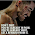pls send me the synthesisable code.my email id is malooksingh84@ymail.com

21.I want to write VHDL code for RADIX 4 FFT algorithm.Can any one help me out.

22.please send me the synthesizable code to my email : bikeforearth@gmail.com, thanks in advance

23.i wanted a vhdl code for 256 point fft.Can anybody pls help me..if anybody has the code can u mail it to alvinmtixon@gmail.com

24.pls send 8 point fft synthesizeable code for xilinx spartan 3e to rishkansonal@gmail.com

25.please can u send me synthesizebable code? if u have in verilog it vl be much useful.. please... can u mail it to sunkarapavani1@gmail.com

26.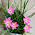hi working 128 point fft processor design using schematic in xilinx can any one give me the vhdl code for tht

27.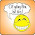please send me the synthesizable code to my email : nvphe1905@gmail.com, thank you:)

28.Please send me the synthesizable code to my email : hdo.altamar@gmail.com, thank you

29.please send me the synthesizable code to my email :pravallika.ulchi@gmail.com

30.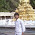please send me the synthesis code

31.Hi...can anyone please send me the synthesizable vhdl code? vinos.mars@gmail.com

32.Hello..I have this error could you tell me why,please?
g2<=sub(s1,mult(s2,w));
ERROR:Xst:1567 - "C:/.Xilinx/fft/fft.vhd" line 127: Signal of type real is not supported.

33.module rt1(x,y);
input [3:0]x;
output [2:0]y;
reg[2:0]y;
always @(x)
begin
case(1'b1)
x: y=4;
x: y=6;
x: y=8;
x: y=2;
endcase
end
endmodule
is it synthesis -able?

34.Im getting no compilation error, but simulation is not processing

35.may i get synthesizable code for ths
my emaid id: jyotizanjurne2@gmail.com

36.may i get synthesizable code for this
My email id:mounikasure060@gmail.com

37.Hi
I want to RTL view for the same code . How can i...

38.Please give me synthesizable code to annanujoseph@gmail.com
Also, can you send me the code to find 2D FFT?